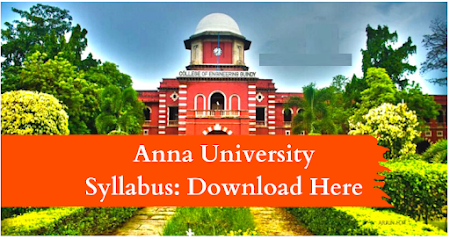### EC8251     CIRCUIT ANALYSIS  L T P C 4 0 0 4

OBJECTIVES:
 To introduce the basic concepts of DC and AC circuits behavior  To study the transient and steady state response of the circuits subjected to step and sinusoidal excitations.  To introduce different methods of circuit analysis using Network theorems, duality and topology.

UNIT I        BASIC CIRCUITS ANALYSIS AND NETWORK TOPOLOGY                        12 Ohm‘s Law – Kirchhoff‘s laws – Mesh current and node voltage method of analysis for D.C and A.C. circuits - Network terminology - Graph of a network - Incidence and reduced incidence matrices – Trees –Cutsets - Fundamental cutsets - Cutset matrix – Tie sets - Link currents and Tie set schedules -Twig voltages and Cutset schedules, Duality and dual networks.

UNIT II      NETWORK THEOREMS FOR DC AND AC CIRCUITS                                   12 Network theorems -Superposition theorem, Thevenin‘s theorem, Norton‘s theorem, Reciprocity theorem, Millman‘s theorem, and Maximum power transfer theorem ,application of Network theorems- Network reduction: voltage and current division, source transformation – star delta conversion.

UNIT III    RESONANCE AND COUPLED CIRCUITS                                                           12 Resonance - Series resonance - Parallel resonance - Variation of impedance with frequency - Variation in current through and voltage across L and C with frequency – Bandwidth - Q factor - Selectivity. Self inductance - Mutual inductance - Dot rule - Coefficient of coupling - Analysis of multiwinding coupled circuits - Series, Parallel connection of coupled inductors - Single tuned and double tuned coupled circuits.

UNIT IV    TRANSIENT ANALYSIS                                                                                           12 Natural response-Forced response - Transient response of RC, RL and RLC circuits to excitation by Step Signal, Impulse Signal and exponential sources - Complete response of RC, RL and RLC Circuits to sinusoidal excitation.

UNIT V     TWO PORT NETWORKS                                                                                          12 Two port networks, Z parameters, Y parameters, Transmission (ABCD) parameters, Hybrid(H) Parameters, Interconnection of two port networks, Symmetrical properties of T and π networks.

TOTAL : 60 PERIODS
OUTCOEMS: At the end of the course, the student should be able to:  Develop the capacity to analyze electrical circuits, apply the circuit theorems in real time  Design and understand and evaluate the AC and DC circuits.

TEXT BOOKS:
1. William H. Hayt, Jr. Jack E. Kemmerly and Steven M. Durbin, ―Engineering Circuit Analysis‖ , McGraw Hill Science Engineering, Eighth Edition, 11th Reprint 2016.
2. Joseph Edminister and Mahmood Nahvi, ―Electric Circuits‖, Schaum‘s Outline Series, Tata McGraw Hill Publishing Company, New Delhi, Fifth Edition Reprint 2016.

REFERENCES:
1. Charles K. Alexander, Mathew N.O. Sadiku, ―Fundamentals of Electric Circuits‖, Fifth Edition, McGraw Hill, 9th Reprint 2015.
2. A.Bruce Carlson, ―Cicuits: Engineering Concepts and Analysis of Linear Electric Circuits‖, Cengage Learning, India Edition 2nd Indian Reprint 2009.
3. Allan H.Robbins, Wilhelm C.Miller, ―Circuit Analysis Theory and Practice‖, Cengage Learning, Fifth Edition, 1st Indian Reprint 2013.